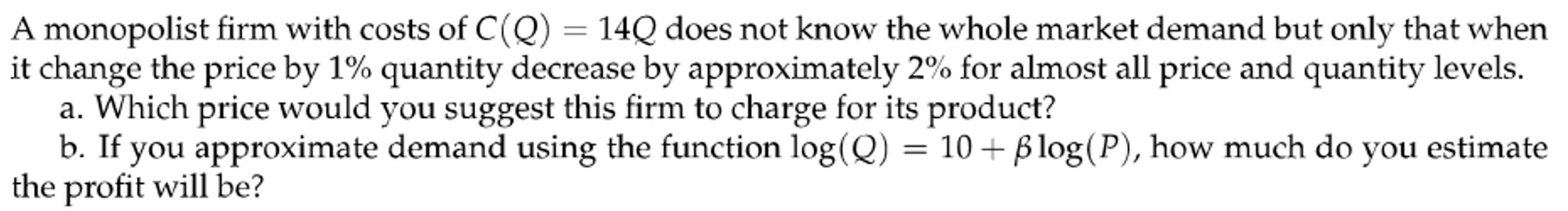Home / Expert Answers / Economics / a-monopolist-firm-with-costs-of-c-q-14-q-does-not-know-the-whole-market-demand-but-only-tha-pa967

# (Solved): A monopolist firm with costs of $$C(Q)=14 Q$$ does not know the whole market demand but only tha ...A monopolist firm with costs of $$C(Q)=14 Q$$ does not know the whole market demand but only that when it change the price by $$1 \%$$ quantity decrease by approximately $$2 \%$$ for almost all price and quantity levels. a. Which price would you suggest this firm to charge for its product? b. If you approximate demand using the function $$\log (Q)=10+\beta \log (P)$$, how much do you estimate the profit will be?

We have an Answer from Expert